www.dlr.de · Antonin RAFFIN · RL on real robots · RL Dresden · 15.09.2022

### Training RL agents directly on real robots

Antonin RAFFIN ( @araffin2 )
German Aerospace Center (DLR)
https://araffin.github.io/

#### Who am I?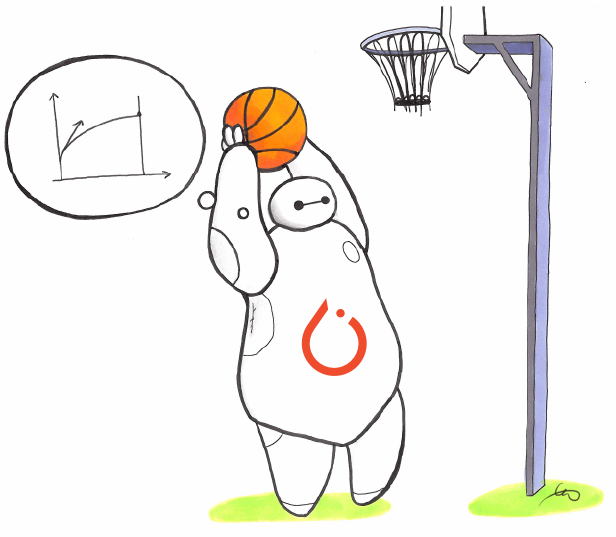Stable-Baselines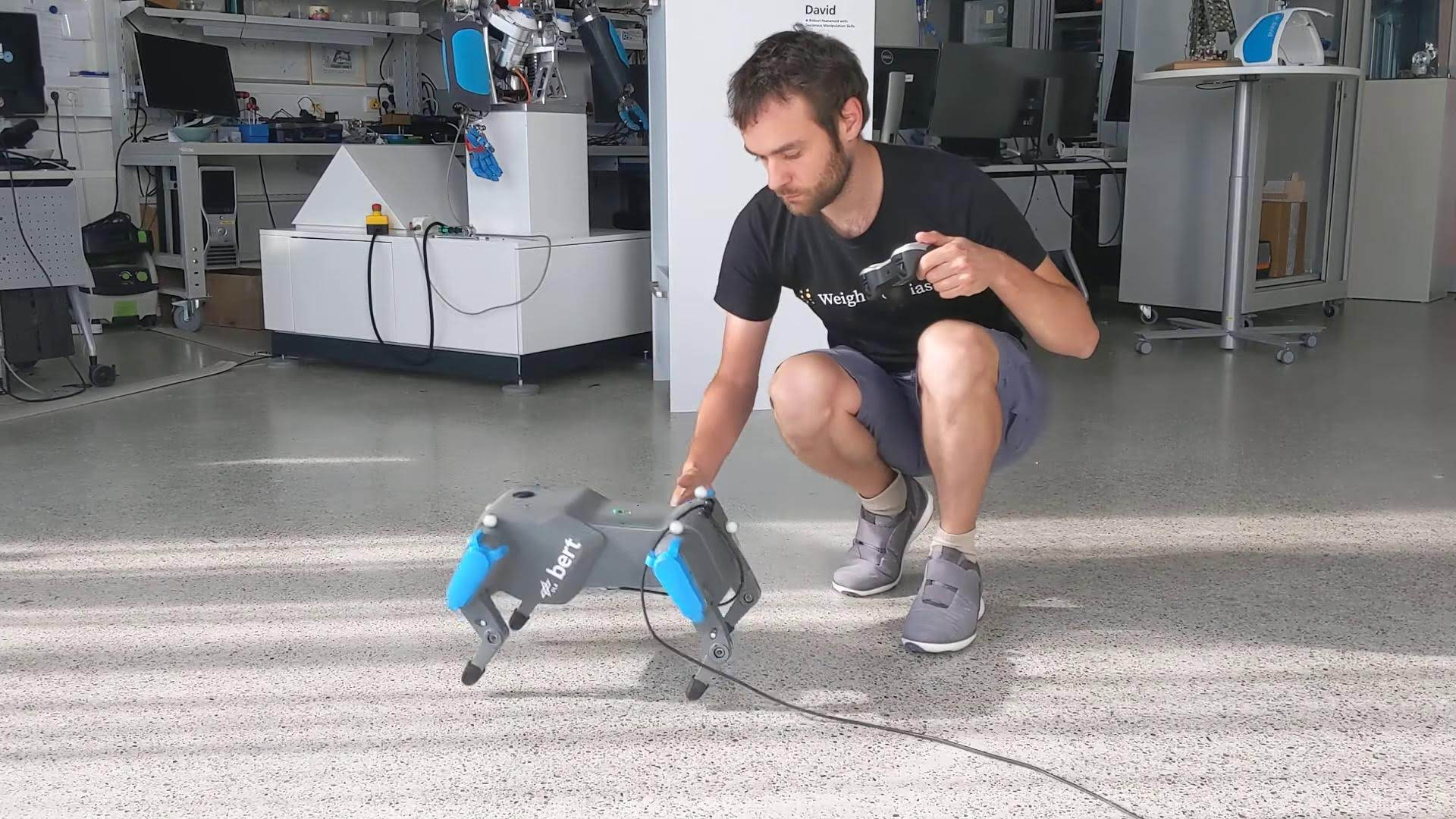bert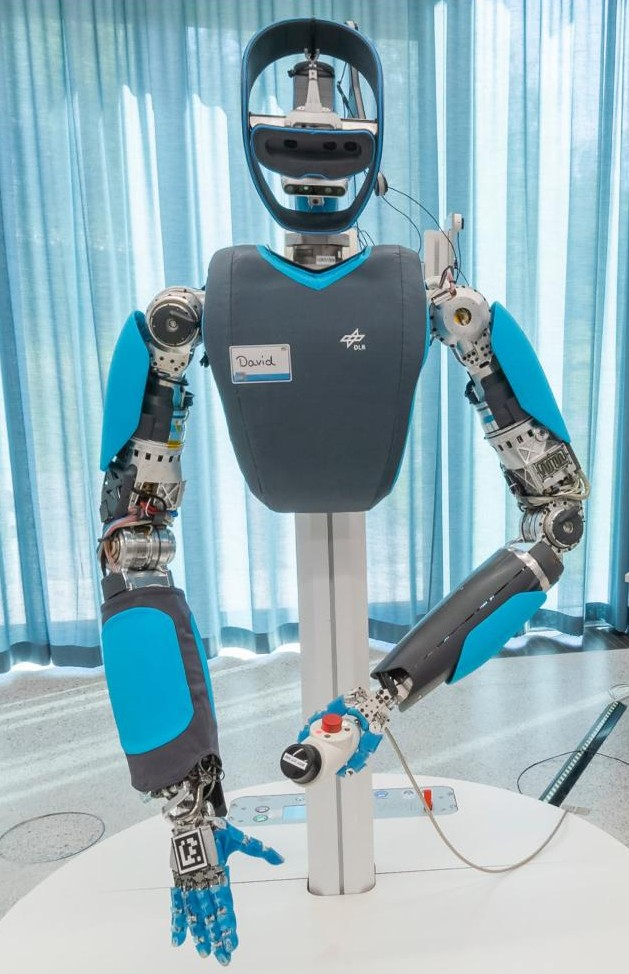David (aka HASy)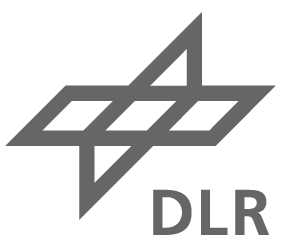German Aerospace Center (DLR)

### Outline

1. Why learn directly on real robots?
2. Learning from scratch
3. Knowledge guided RL
4. Questions?

### Why learn directly on real robots?

Miki, Takahiro, et al. "Learning robust perceptive locomotion for quadrupedal robots in the wild." Science Robotics (2022)

Rudin, Nikita, et al. "Learning to walk in minutes using massively parallel deep reinforcement learning." CoRL. PMLR, 2022.

### Simulation is all you need?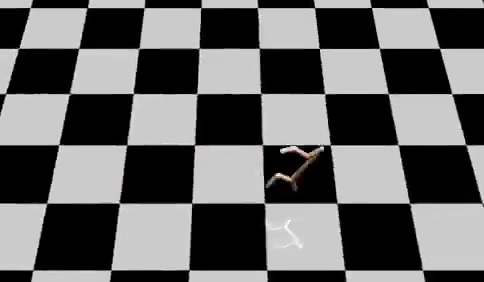Credits: Nathan Lambert (@natolambert)

### Why learn directly on real robots?

• because you can! (software/hardware)
• simulation is safer, faster
• simulation to reality (sim2real): accurate model and randomization needed
• challenges: robot safety, sample efficiency

### Learning from scratch

#### Learning to control an elastic robot

##### Challenges
• hard to model (silicon neck)
• oscillations
• 2h on the real robot (safety)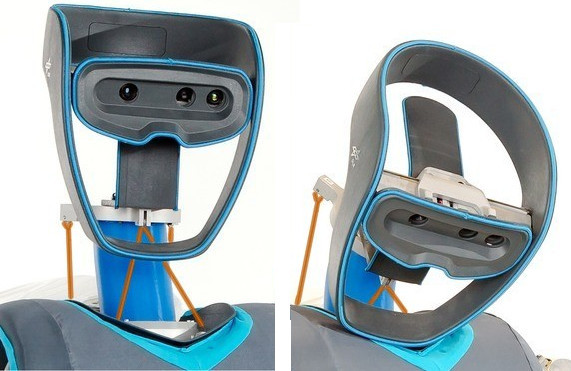Raffin, Antonin, Jens Kober, and Freek Stulp. "Smooth exploration for robotic reinforcement learning." CoRL. PMLR, 2022.

#### Smooth Exploration for Robotic RL

Independent Gaussian noise: $\epsilon_t \sim \mathcal{N}(0, \sigma)$ $a_t = \mu(s_t; \theta_{\mu}) + \epsilon_t$
State dependent exploration: $\theta_{\epsilon} \sim \mathcal{N}(0, \sigma_{\epsilon})$ $a_t = \mu(s_t; \theta_{\mu}) + \epsilon(s_t; \theta_{\epsilon})$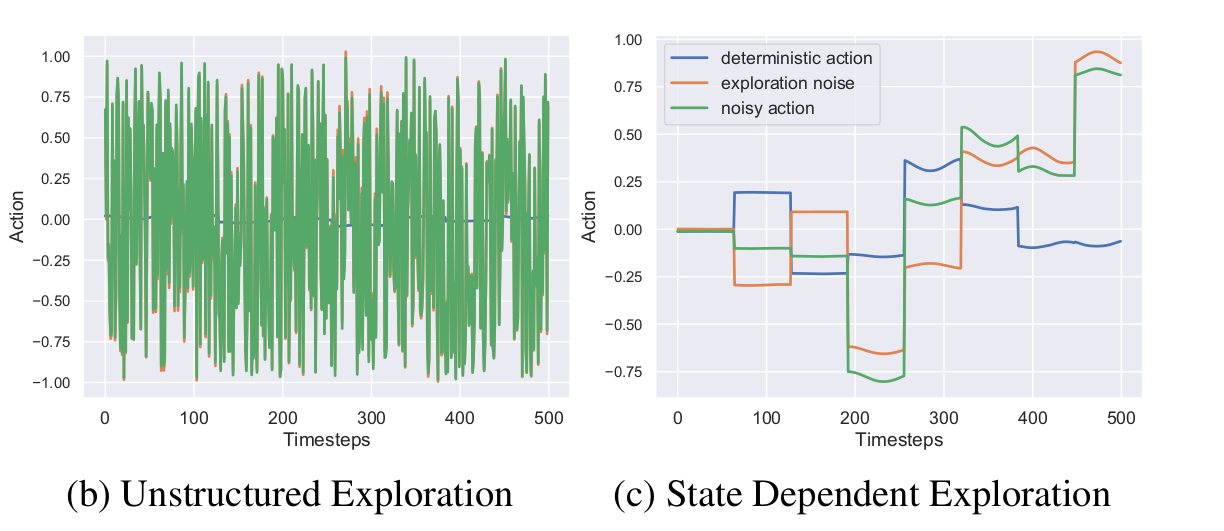#### Learning to walk with an elastic quadruped robot

##### Challenges
• robot safety (5h+ of training)
• manual reset
• communication delay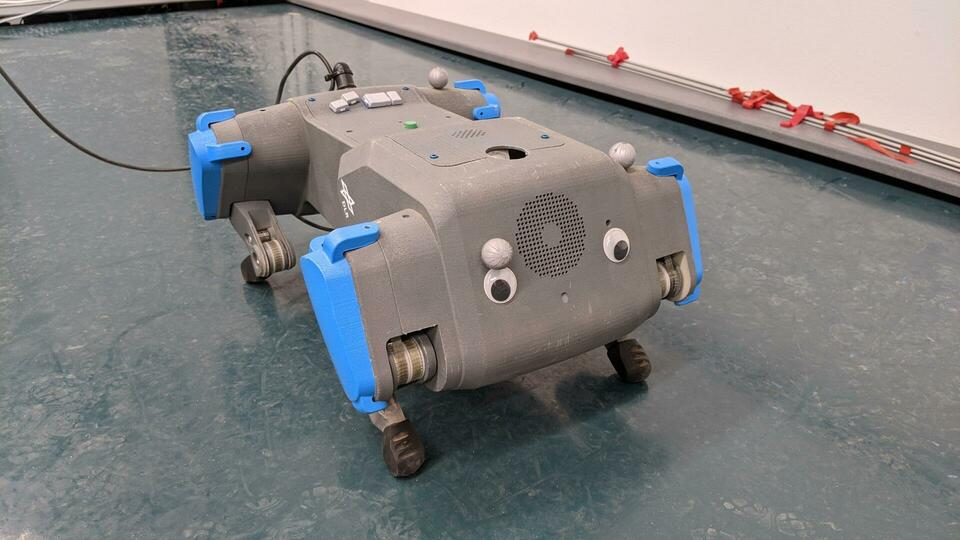Raffin, Antonin, Jens Kober, and Freek Stulp. "Smooth exploration for robotic reinforcement learning." CoRL. PMLR, 2022.

#### DroQ - 20 Minutes Training

Smith, Laura, Ilya Kostrikov, and Sergey Levine. "A Walk in the Park: Learning to Walk in 20 Minutes With Model-Free Reinforcement Learning." arXiv preprint (2022).

### Knowledge guided RL

• knowledge about the robot (neck)
• RL for improved robustness (CPG + RL)

#### Learning to drive in minutes / learning to race in hours

##### Challenges
• minimal number of sensors (image, speed)
• variability of the scene (light, shadows, other cars, ...)
• limited computing power
• communication delay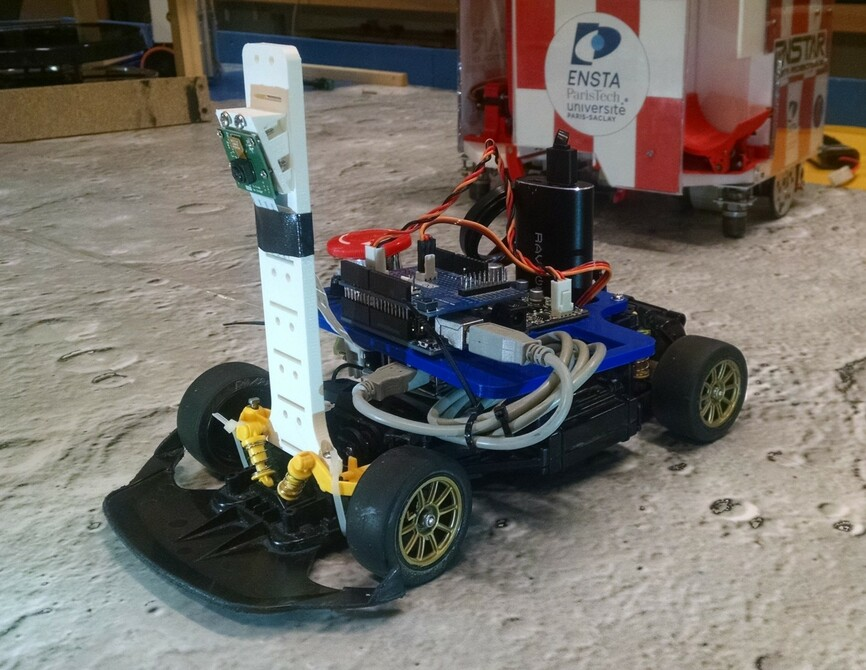Raffin, Antonin, Jens Kober, and Freek Stulp. "Smooth exploration for robotic reinforcement learning." CoRL. PMLR, 2022.

#### Learning a state representation (SRL)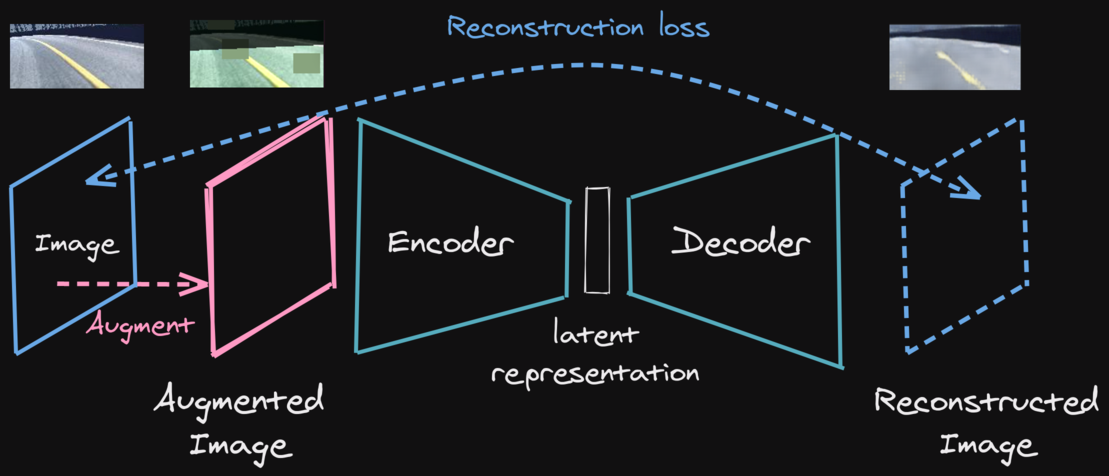#### Pre-trained agent on Huggingface hub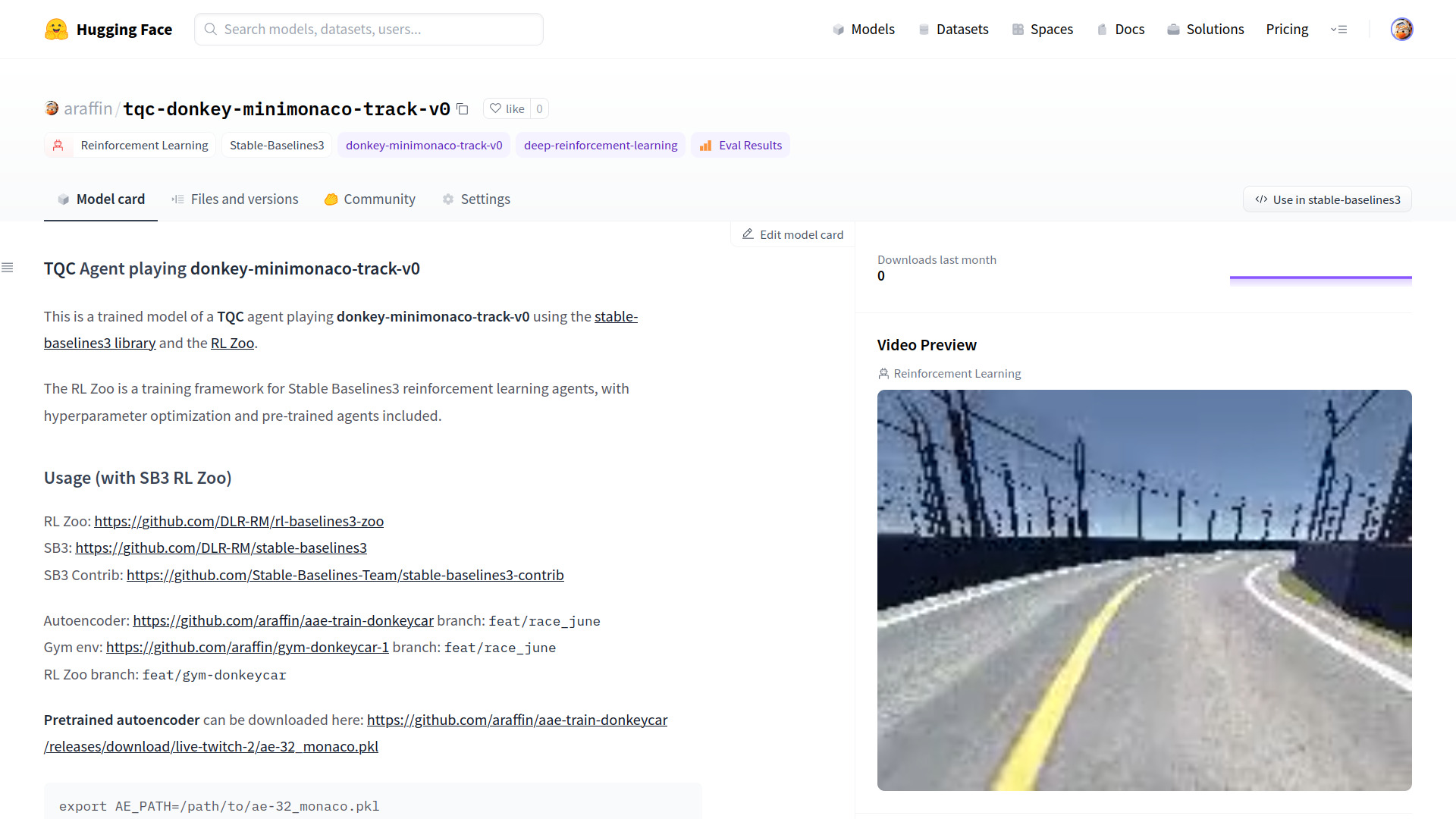#### Learning to Exploit Elastic Actuators for Quadruped LocomotionRaffin et al. "Learning to Exploit Elastic Actuators for Quadruped Locomotion" In preparation ICRA, 2023.

#### Otimized CPG + RL

Coupled oscillator

\begin{aligned} \dot{r_i} & = a (\mu - r_i^2)r_i \\ \dot{\varphi_i} & = \omega + \sum_j \, r_j \, c_{ij} \, \sin(\varphi_j - \varphi_i - \Phi_{ij}) \\ \end{aligned}Desired foot position

\begin{aligned} x_{des,i} &= \textcolor{#5f3dc4}{\Delta x_\text{len}} \cdot r_i \cos(\varphi_i)\\ z_{des,i} &= \Delta z \cdot \sin(\varphi_i) \\ \Delta z &= \begin{cases} \textcolor{#5c940d}{\Delta z_\text{clear}} &\text{if \sin(\varphi_i) > 0 (\textcolor{#0b7285}{swing})}\\ \textcolor{#d9480f}{\Delta z_\text{pen}} &\text{otherwise (\textcolor{#862e9c}{stance}).} \end{cases} \end{aligned}

closing the loop with RL

\begin{aligned} x_{des,i} &= \textcolor{#a61e4d}{\Delta x_\text{len} \cdot r_i \cos(\varphi_i)} + \textcolor{#1864ab}{\pi_{x,i}(s_t)} \\ z_{des,i} &= \textcolor{#a61e4d}{\Delta z \cdot \sin(\varphi_i)} + \textcolor{#1864ab}{\pi_{z,i}(s_t)} \end{aligned}

### Fast Trot (~30 minutes training)

#### Learning to Exploit Elastic Actuators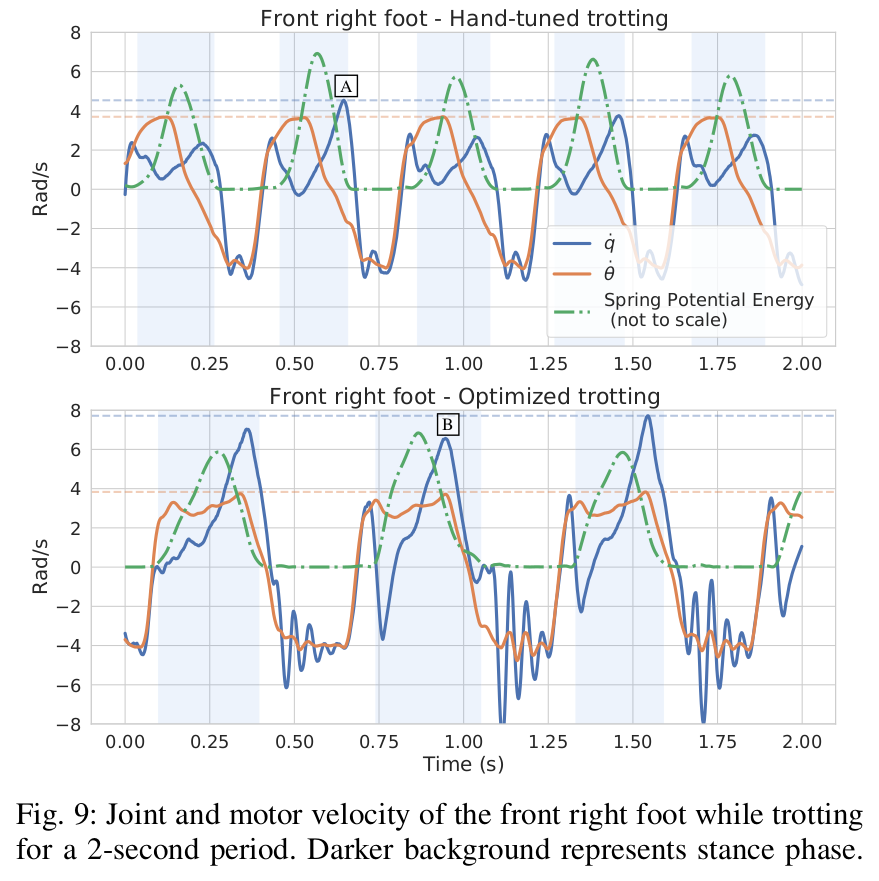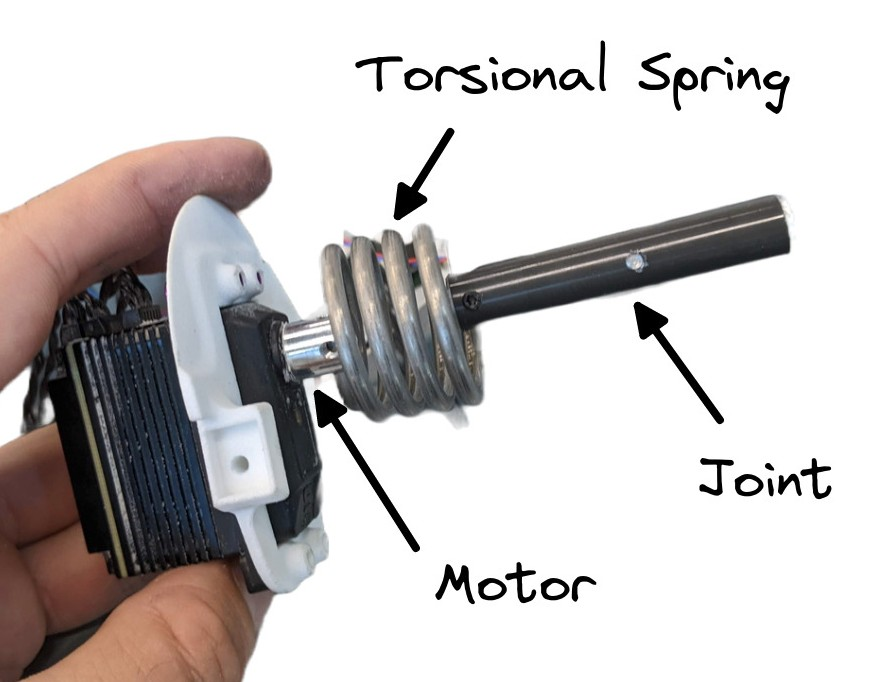#### Patterns#### Recap

• simulation is all you need
• learning directly on a real robot is possible
• knowledge guided RL to improve efficiency

### Questions?

• formulation: $r_{continuity} = - (a_t - a_{t - 1})^2$